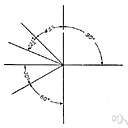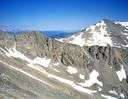# vertex

(redirected from vertexes)
Also found in: Thesaurus, Medical, Encyclopedia.
Related to vertexes: vertices

## ver·tex

(vûr′tĕks′)
n. pl. ver·ti·ces (-tĭ-sēz′) also ver·tex·es
1. The highest point; the apex or summit: the vertex of a mountain.
2. Anatomy
a. The highest point of the skull.
b. The top of the head.
3. In astrology, the highest point reached in the apparent motion of a celestial body.
4. Mathematics
a. The point at which the sides of an angle intersect.
b. The point on a triangle or pyramid opposite to and farthest away from its base.
c. A point on a polyhedron common to three or more sides.
d. A point of maximal curvature on a parabola or hyperbola.

[Latin, whirlpool, crown of the head (where the hair forms a whorl), vertex, from vertere, to turn; see wer- in Indo-European roots.]

## vertex

(ˈvɜːtɛks)
n, pl -texes or -tices (-tɪˌsiːz)
1. the highest point
2. (Mathematics) maths
a. the point opposite the base of a figure
b. the point of intersection of two sides of a plane figure or angle
c. the point of intersection of a pencil of lines or three or more planes of a solid figure
3. (Astronomy) astronomy a point in the sky towards which a star stream appears to move
4. (Anatomy) anatomy the crown of the head
[C16: from Latin: highest point, from vertere to turn]

## ver•tex

(ˈvɜr tɛks)

n., pl. -tex•es, -ti•ces (-təˌsiz)
1. the highest point; apex.
2. the top of the head.
3. a point in the celestial sphere toward which or from which the common motion of a group of stars is directed.
4. Geom.
a. the point farthest from the base.
b. a point in a geometrical solid common to three or more sides.
c. the intersection of two sides of a plane figure.
[1560–70; < Latin: whirl, top (of the head), derivative of vert(ere) to turn]

## ver·tex

(vûr′tĕks′)
Plural vertices (vûr′tĭ-sēz′) or vertexes
1. The point at which the sides of an angle intersect.
2. The point of a triangle, cone, or pyramid that is opposite to and farthest away from its base; the apex.
3. A point of a polyhedron at which three or more of the edges intersect.

## vertex

In artillery and naval gunfire support, the highest point in the trajectory of a projectile.
ThesaurusAntonymsRelated WordsSynonymsLegend:
 Noun 1vertex - the point of intersection of lines or the point opposite the base of a figureintersection, intersection point, point of intersection - a point where lines intersect 2vertex - the highest point (of something); "at the peak of the pyramid"crown - the part of a hat (the vertex) that covers the crown of the headroof peak - the highest point of a roofextreme point, extremum, extreme - the point located farthest from the middle of something

## vertex

noun the vertex of the triangle

## vertex

noun
The highest point:
Translations
vrchol
huippukärkikeskipistepäälakisolmu
verteksvrh
viršugalvisviršūnė

## vertex

[vɜːteks] N (vertexes or vertices (pl)) [vɜːtɪsiːz] (Math, Archit) →

## vertex

n pl <vertices> → Scheitel(punkt) m

## vertex

[ˈvɜːtɛks] n (vertices (pl)) [ˈvɜːtɪsiːz]

## ver·tex

n. vértice.
1. cúspide de una estructura, tal como el punto extremo de la cabeza;
2. punto en que concurren los lados de un ángulo.

## vertex

n (anat) coronilla, vértice m, parte f superior de la cabeza
References in periodicals archive ?
Assign vertices codes of A and A' in two groups: the hollow vertexes to be the first group and the solid vertexes to be another group.
Multiple VertExes can be used to create large extracted booths, or single units can be used directly behind an operator to allow at-source extraction from dusty processes.
Then [v.sub.k + 1] and [v.sub.k + 2] are its antipodal vertices at a distance k from [v.sub.1] and there is no geodesic path from the vertexes [v.sub.k + 1] and [v.sub.k + 2] to any other vertex passing through [v.sub.1].

Site: Follow: Share:
Open / Close